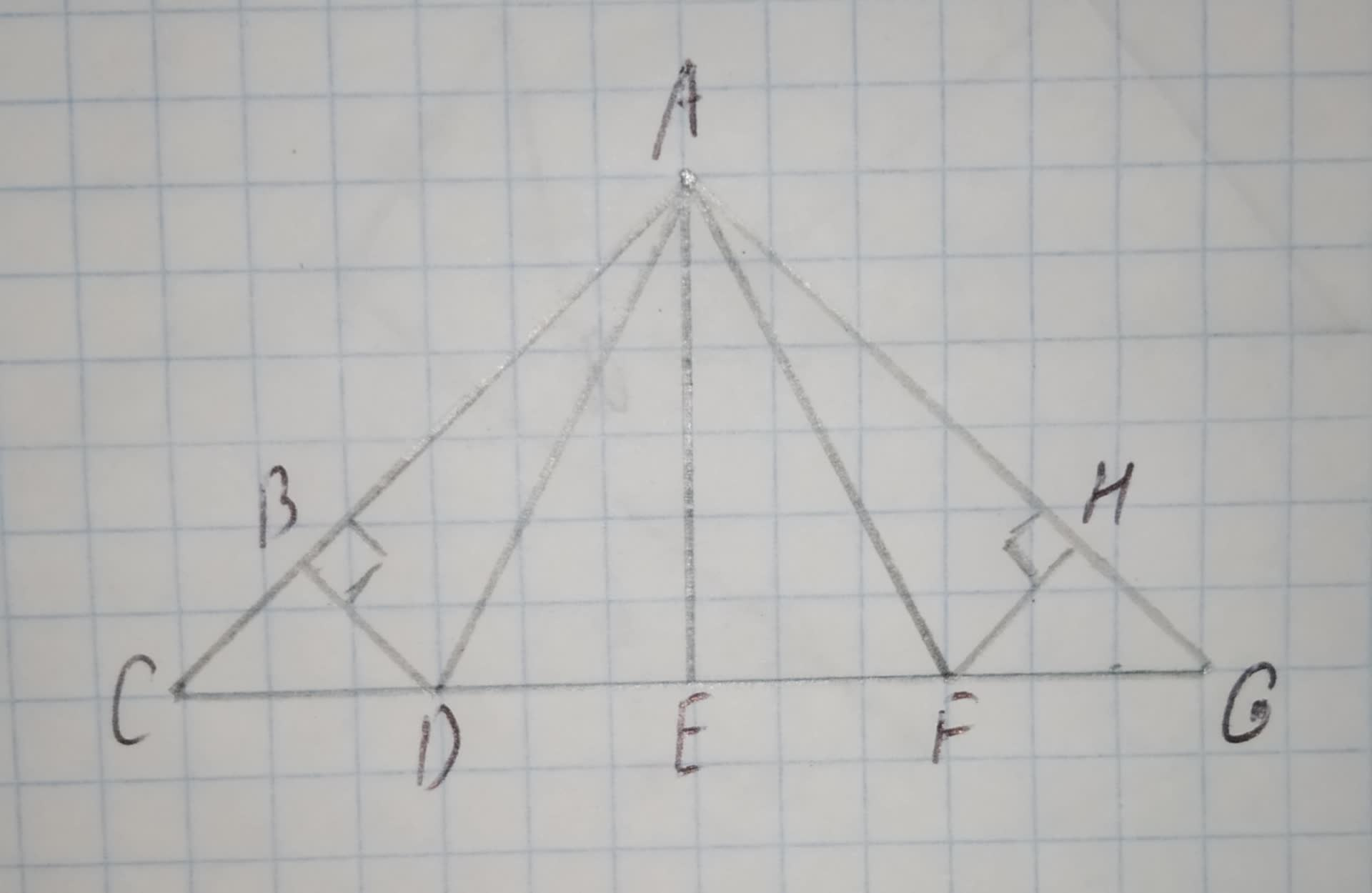# In the roof truss shown, AB = 8 and m/_HAF = 37^(circ). Find: AH. (HINT: The design of the roof truss displays line symmetry.) 12210203661.jpgCem Hayes 2020-12-24 Answered
In the roof truss shown, AB = 8 and $m\mathrm{\angle }HAF={37}^{\circ }$. Find: AH.
(HINT: The design of the roof truss displays line symmetry.)You can still ask an expert for help

## Want to know more about Congruence?

• Questions are typically answered in as fast as 30 minutes

Solve your problem for the price of one coffee

• Math expert for every subject
• Pay only if we can solve itElberte
Step 1
Find the value of AH.
Step 2
The roof truss has line symmetry about line $\stackrel{\to }{AE}$
So $\mathrm{△}ABD\stackrel{\sim }{=}\mathrm{△}AHF$
Use transitive property of congruence.
AH and HB are the sides of the congruent triangles, AH=AB.
Substitute AB=8 in AH=AB.
AH=8
Hence, the value of AH is 8.